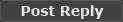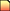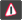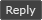(2 Kb)
Updated: 09-20-16 02:56 PM
Pictures
File Info
 Updated: 09-20-16 02:56 PM Created: 09-20-16 02:56 PM Downloads: 1,795 Favorites: 9 MD5: Categories: Chat Mods, Discontinued and Outdated Mods

# bdBetterChatBubbles

Version: 1
by: Blooblahguy [More]

bdBetterChatBubbles makes it so that player name as well as any spell or item links show up in chat bubbles.

note: You need bdCore to use this addon, or any other bd_ addons

Features

Shows player name in chat bubble
Shows spell/item links in chat bubbles
Configuration to skin/remove bubble background
/bd config to access in game config
/bd lock to unlock the addon and move it around

NOTE: Some other chat mods may break this depending on what they change about the chat log. This works with bdChat and default UI. May vary with other chat mods.

Optional Files (0)Comment Options03-10-17, 11:13 AM Suiche A Kobold Labourer Forum posts: 0 File comments: 13 Uploads: 0 Downloaded the whole set of addons you made... Amazing work BTW! Here is the ChatBubble Error. 617x bdBetterChatBubbles\core.lua:32: attempt to get length of field 'chatstrings' (a nil value) bdBetterChatBubbles\core.lua:32: in function (tail call): ? FrameXML\ChatFrame.lua:3246: in function `ChatFrame_MessageEventHandler' FrameXML\ChatFrame.lua:2674: in function (tail call): ? [string "*:OnEvent"]:1: in function <[string "*:OnEvent"]:1> Locals: frame = ChatFrame1 { 0 = RefreshIfNecessary = defined =[C]:-1 OnPostShow = defined =[C]:-1 isInitialized = 1 GetNumVisibleLines = defined =[C]:-1 ScrollUp = defined =[C]:-1 SetTimeVisible = defined =[C]:-1 hasOwnFontObject = true OnFontObjectUpdated = defined =[C]:-1 resizeButton = ChatFrame1ResizeButton { } GetFading = defined =[C]:-1 Clear = defined =[C]:-1 shouldFadeAfterInactivity = false SetIndentedWordWrap = defined =[C]:-1 GetTimeVisible = defined =[C]:-1 GetJustifyV = defined =[C]:-1 SetFont = defined =[C]:-1 DefaultAddMessage = defined @bdBetterChatBubbles\core.lua:21 GetMaxLines = defined =[C]:-1 Show = defined =[C]:-1 BackFillMessage = defined =[C]:-1 UnpackageEntry = defined =[C]:-1 FontStringContainer = { } SetShadowOffset = defined =[C]:-1 messageTypeList = { } GetFont = defined =[C]:-1 checkedGMOTD = true SetMaxLines = defined =[C]:-1 timeVisibleSecs = 120 PageUp = defined =[C]:-1 RefreshDisplay = defined =[C]:-1 SetFontObject = defined =[C]:-1 MakeFontObjectCustom = defined =[C]:-1 CalculateNumVisibleLines = defined =[C]:-1 fontObject = table: 000001FE8D1D2510 { } overrideFadeTimestamp = 100433.928 SetInsertMode = defined =[C]:-1 MarkDisplayDirty = defined =[C]:-1 mouseInTime = 0 GetMessageInfo = defined =[C]:-1 ScrollDown = defined =[C]:-1 AtTop = defined =[C]:-1 GetFontObject = defined =[C]:-1 zoneChannelList = { } SetScrollOffset = defined =[C]:-1 OnPostUpdate = defined =[C]:-1 fontStringPool = { } channelList = { } buttonSide = "left" CanEffectivelyFade = defined =[C]:-1 ScrollToBottom = defined =[C]:-1 GetInsertMode = defined =[C]:-1 insertMode = 2 oldAlpha = 0 OnPreSizeChanged = defined =[C]:-1 fadeDurationSecs = 3 GetScrollOffset = defined =[C]:-1 isDocked = 1 GetIndentedWordWrap = defined =[C]:-1 alternativeDefaultLanguage = "Orcish" defaultLanguage = "Orcish" Hide = defined =[C]:-1 GetTextColor = defined =[C]:-1 tellTimer = 100401.69 ResetAllFadeTimes = defined =[C]:-1 flashTimer = 0.33800039999187 isDisplayDirty = false GetSpacing = defined =[C]:-1 OnPreLoad = defined =[C]:-1 visibleLines = { } PackageEntry = defined =[C]:-1 SetSpacing = defined =[C]:-1 name = "General" ScrollToTop = defined =[C]:-1 historyBuffer = { } isLayoutDirty = false AdjustMessageColors = defined =[C]:-1 GetNumMessages = defined =[C]:-1 RefreshLayout = defined =[C]:-1 GetShadowColor = defined =[C]:-1 isUninteractable = false AddMessage = defined @bdChat\core.lua:218 GetJustifyH = defined =[C]:-1 mouseOutTime = 0.016000000759959 PageDown = defined =[C]:-1 GetMaxScrollRange = defined =[C]:-1 SetJustifyH = defined =[C]:-1 SetFadeDuration = defined =[C]:-1 GetShadowOffset = defined =[C]:-1 GetPagingScrollAmount = defined =[C]:-1 SetTextColor = defined =[C]:-1 AtBottom = defined =[C]:-1 buttonFrame = ChatFrame1ButtonFrame { } SetShadowColor = defined =[C]:-1 CalculateLineSpacing = defined =[C]:-1 RemoveMessagesByPredicate = defined =[C]:-1 InitializeFontableFrame = defined =[C]:-1 HasFontObject = defined =[C]:-1 clickAnywhereButton = ChatFrame1ClickAnywhereButton { } isStaticDocked = true SetJustifyV = defined =[C]:-1 editBox = ChatFrame1EditBox { } CalculateLineAlphaValueFromTimestamp = <02-14-17, 01:21 PM benzy A Defias Bandit Forum posts: 3 File comments: 66 Uploads: 0 Hey Im getting this lua error quite often m any idea from what it can ? I don't other addons excepts yours . Message: Interface\AddOns\bdBetterChatBubbles\core.lua:32: attempt to get length of field 'chatstrings' (a nil value) Time: 02/14/17 21:01:02 Count: 8 Stack: Interface\AddOns\bdBetterChatBubbles\core.lua:32: in function (tail call): ? Interface\FrameXML\ChatFrame.lua:3246: in function `ChatFrame_MessageEventHandler' Interface\FrameXML\ChatFrame.lua:2674: in function `ChatFrame_OnEvent' [string "*:OnEvent"]:1: in function <[string "*:OnEvent"]:1> Locals: frame = ChatFrame1 { 0 = RefreshIfNecessary = defined =[C]:-1 OnPostShow = defined =[C]:-1 isInitialized = 1 GetNumVisibleLines = defined =[C]:-1 ScrollUp = defined =[C]:-1 SetTimeVisible = defined =[C]:-1 hasOwnFontObject = true OnFontObjectUpdated = defined =[C]:-1 resizeButton = ChatFrame1ResizeButton { } GetFading = defined =[C]:-1 Clear = defined =[C]:-1 shouldFadeAfterInactivity = false SetIndentedWordWrap = defined =[C]:-1 GetTimeVisible = defined =[C]:-1 GetJustifyV = defined =[C]:-1 SetFont = defined =[C]:-1 DefaultAddMessage = defined @Interface\AddOns\bdBetterChatBubbles\core.lua:21 GetMaxLines = defined =[C]:-1 Show = defined =[C]:-1 BackFillMessage = defined =[C]:-1 UnpackageEntry = defined =[C]:-1 FontStringContainer = { } SetShadowOffset = defined =[C]:-1 messageTypeList = { } GetFont = defined =[C]:-1 checkedGMOTD = true SetMaxLines = defined =[C]:-1 timeVisibleSecs = 120 PageUp = defined =[C]:-1 RefreshDisplay = defined =[C]:-1 SetFontObject = defined =[C]:-1 MakeFontObjectCustom = defined =[C]:-1 CalculateNumVisibleLines = defined =[C]:-1 fontObject = table: 000001C79CF8E900 { } overrideFadeTimestamp = 0 SetInsertMode = defined =[C]:-1 MarkDisplayDirty = defined =[C]:-1 mouseInTime = 0 GetMessageInfo = defined =[C]:-1 OnPostUpdate = defined =[C]:-1 alternativeDefaultLanguage = "Common" ScrollDown = defined =[C]:-1 AtTop = defined =[C]:-1 GetFontObject = defined =[C]:-1 zoneChannelList = { } SetScrollOffset = defined =[C]:-1 defaultLanguage = "Common" fontStringPool = { } channelList = { } buttonSide = "left" CanEffectivelyFade = defined =[C]:-1 ScrollToBottom = defined =[C]:-1 GetInsertMode = defined =[C]:-1 insertMode = 2 oldAlpha = 0.15686275064945 OnPreSizeChanged = defined =[C]:-1 fadeDurationSecs = 3 GetScrollOffset = defined =[C]:-1 isDocked = 1 GetIndentedWordWrap = defined =[C]:-1 Hide = defined =[C]:-1 GetTextColor = defined =[C]:-1 tellTimer = 47223.232 ResetAllFadeTimes = defined =[C]:-1 flashTimer = 0 isDisplayDirty = false GetSpacing = defined =[C]:-1 OnPreLoad = defined =[C]:-1 visibleLines = { } PackageEntry = defined =[C]:-1 SetSpacing = defined =[C]:-1 name = "General" ScrollToTop = defined =[C]:-1 historyBuffer = { } isLayoutDirty = false AdjustMessageColors = defined =[C]:-1 GetNumMessages = defined =[C]:-1 RefreshLayout = defined =[C]:-1 GetShadowColor = defined =[C]:-1 isUninteractable = false AddMessage = defined @Interface\AddOns\bdChat\core.lua:216 GetJustifyH = defined =[C]:-1 mouseOutTime = 0.010000000707805 PageDown = defined =[C]:-1 GetMaxScrollRange = defined =[C]:-1 SetJustifyH = defined =[C]:-1 SetFadeDuration = defined =[C]:-1 GetShadowOffset = defined =[C]:-1 GetPagingScrollAmount =Report this File

Category Jump: